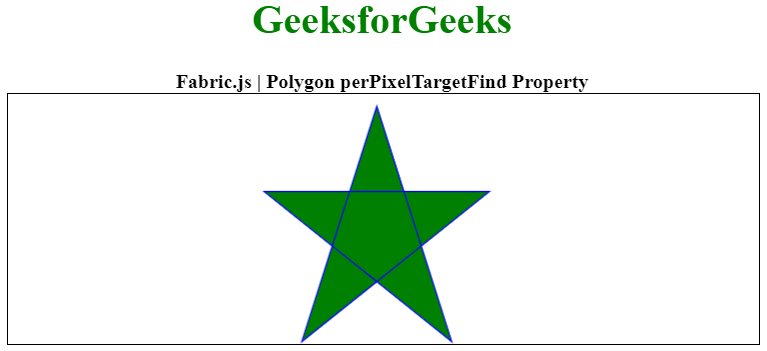Related Articles

# Fabric.js Polygon perPixelTargetFind Property

• Last Updated : 20 Jan, 2021

In this article, we are going to see the perPixelTargetFind property of a polygon canvas using FabricJS. The canvas polygon means the polygon is movable and can be stretched according to requirements. Further, the polygon can be customized when it comes to initial stroke color, height, width, fill color, or stroke width.

To make it possible we are going to use a JavaScript library called FabricJS. After importing the library, we will create a canvas block in the body tag that will contain the polygon. After this, we will initialize instances of Canvas and polygon provided by FabricJS and set the perPixelTargetFind of canvas polygon using perPixelTargetFind property, and render the polygon on the Canvas as given in the below example.

Syntax:

```fabric.Polygon([
{ x: pixel, y: pixel },
{ x: pixel, y: pixel },
{ x: pixel, y: pixel},
{ x: pixel, y: pixel},
{ x: pixel, y: pixel }
],
{
perPixelTargetFind: Boolean
});```

Parameters: This property accepts a single parameter as mentioned above and described below:

• perPixelTargetFind: When set to `true`, objects are “found” on canvas on a per-pixel basis rather than according to the bounding box.

Below examples illustrate the Fabric.JS Polygon perPixelTargetFind in JavaScript:

Example:

## HTML

 ` ``<``html``> `` ` `<``head``> ``  ````  ``<``script` `src``= ``"https://cdnjs.cloudflare.com/ajax/libs/fabric.js/3.6.2/fabric.min.js"``> ``  `` `` `` ` `<``body``> ``  ``<``div` `style``=``"text-align: center;width: 600px;"``> ``    ``<``h1` `style``=``"color: green;"``> ``        ``GeeksforGeeks ``    `` ``    ``<``b``> ``        ``Fabric.js | Polygon perPixelTargetFind Property ``    `` ``  `` ``  ``<``canvas` `id``=``"canvas"` `width``=``"600"` `height``=``"200"``      ``style``=``"border:1px solid #000000;"``> ``  `` `` ` `  ``<``script``> ``    ``// Initiate a Canvas instance ``    ``var canvas = new fabric.Canvas("canvas"); `` ` `    ``// Initiate a polygon instance ``    ``var polygon = new fabric.Polygon([ ``        ``{ x: 295, y: 10 }, ``        ``{ x: 235, y: 198 }, ``        ``{ x: 385, y: 78 }, ``        ``{ x: 205, y: 78 }, ``        ``{ x: 355, y: 198 }], {``            ``fill:'green',``            ``stroke: 'blue',``         ``paintFirst: 'fill',``         ``perPixelTargetFind: 'true'`` ` `    ``}); `` ` `    ``// Render the polygon in canvas ``    ``canvas.add(polygon); ``  `` `` `` ` ``

Output:My Personal Notes arrow_drop_up